### Hypothesis Tests for Simple Univariate Models

If the dependent variable has one parameter (IDENTITY, LINEAR with no missing values, and so on) and if there are no monotonicity constraints, PROC TRANSREG fits univariate models, which can also be fit with a DATA step and PROC REG. This is illustrated with the following artificial data set:

data htex;
do i = 0.5 to 10 by 0.5;
x1 = log(i);
x2 = sqrt(i) + sin(i);
x3 = 0.05 * i * i + cos(i);
y  = x1 - x2 + x3 + 3 * normal(7);
x1 = x1 + normal(7);
x2 = x2 + normal(7);
x3 = x3 + normal(7);
output;
end;
run;


Both PROC TRANSREG and PROC REG are run to fit the same polynomial regression model as follows:

proc transreg data=htex ss2 short;
title 'Fit a Polynomial Regression Model with PROC TRANSREG';
model identity(y) = spline(x1);
run;

data htex2;
set htex;
x1_1 = x1;
x1_2 = x1 * x1;
x1_3 = x1 * x1 * x1;
run;

proc reg;
title 'Fit a Polynomial Regression Model with PROC REG';
model y = x1_1 - x1_3;
run; quit;


The ANOVA and regression tables from PROC TRANSREG are displayed in Figure 97.68. The ANOVA and regression tables from PROC REG are displayed in Figure 97.69. The SHORT a-option is specified with PROC TRANSREG to suppress the iteration history.

Figure 97.68: ANOVA and Regression Output from PROC TRANSREG

 Fit a Polynomial Regression Model with PROC TRANSREG

The TRANSREG Procedure

Dependent Variable Identity(y)

 Number of Observations Read 20 20

Identity(y)
Algorithm converged.

The TRANSREG Procedure Hypothesis Tests for Identity(y)

Univariate ANOVA Table Based on the Usual Degrees of Freedom
Source DF Sum of Squares Mean Square F Value Pr > F
Model 3 5.8365 1.94550 0.14 0.9329
Error 16 218.3073 13.64421
Corrected Total 19 224.1438

 Root MSE R-Square 3.69381 0.0260 0.8549 -0.1566 432.073

Univariate Regression Table Based on the Usual Degrees of Freedom
Variable DF Coefficient Type II
Sum of
Squares
Mean Square F Value Pr > F
Intercept 1 1.4612767 18.8971 18.8971 1.38 0.2565
Spline(x1) 3 -0.3924013 5.8365 1.9455 0.14 0.9329

Figure 97.69: ANOVA and Regression Output from PROC REG

 Fit a Polynomial Regression Model with PROC REG

The REG Procedure
Model: MODEL1
Dependent Variable: y

 Number of Observations Read 20 20

Analysis of Variance
Source DF Sum of
Squares
Mean
Square
F Value Pr > F
Model 3 5.83651 1.94550 0.14 0.9329
Error 16 218.30729 13.64421
Corrected Total 19 224.14380

 Root MSE R-Square 3.69381 0.0260 0.8549 -0.1566 432.073

Parameter Estimates
Variable DF Parameter
Estimate
Standard
Error
t Value Pr > |t|
Intercept 1 1.22083 1.47163 0.83 0.4190
x1_1 1 0.79743 1.75129 0.46 0.6550
x1_2 1 -0.49381 1.50449 -0.33 0.7470
x1_3 1 0.04422 0.32956 0.13 0.8949

The PROC TRANSREG regression table differs in several important ways from the parameter estimate table produced by PROC REG. The REG procedure displays standard errors and t statistics. PROC TRANSREG displays Type II sums of squares, mean squares, and F statistics. The difference is because the numerator degrees of freedom are not always 1, so t tests are not uniformly appropriate. When the degrees of freedom for variable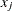is 1, the following relationships hold between the standard errors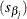and the Type II sums of squares ():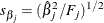and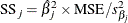PROC TRANSREG does not provide tests of the individual terms that go into the transformation. (However, it could if BSPLINE or PSPLINE had been specified instead of SPLINE.) The test of spline(x1) is the same as the test of the overall model. The intercepts are different due to the different numbers of variables and their standardizations.

In the next example, both x1 and x2 are transformed in the first PROC TRANSREG step, and PROC TRANSREG is used instead of a DATA step to create the polynomials for PROC REG. Both PROC TRANSREG and PROC REG fit the same polynomial regression model. The following statements run PROC TRANSREG and PROC REG and produce Figure 97.70 and Figure 97.71:

title 'Two-Variable Polynomial Regression';

proc transreg data=htex ss2 solve;
model identity(y) = spline(x1 x2);
run;

proc transreg noprint data=htex maxiter=0;
/* Use PROC TRANSREG to prepare input to PROC REG */
model identity(y) = pspline(x1 x2);
output out=htex2;
run;

proc reg data=htex2;
model y = x1_1-x1_3 x2_1-x2_3;
test x1_1, x1_2, x1_3;
test x2_1, x2_2, x2_3;
run; quit;


Figure 97.70: Two-Variable Polynomial Regression Output from PROC TRANSREG

 Two-Variable Polynomial Regression

The TRANSREG Procedure

Dependent Variable Identity(y)

 Number of Observations Read 20 20

TRANSREG MORALS Algorithm Iteration History for Identity(y)
Iteration
Number
Average
Change
Maximum
Change
R-Square Criterion
Change
Note
0 0.69502 4.73421 0.08252
1 0.00000 0.00000 0.17287 0.09035 Converged

 Algorithm converged.

Hypothesis Test Iterations Excluding Spline(x1)
TRANSREG MORALS Algorithm Iteration History for Identity(y)
Iteration
Number
Average
Change
Maximum
Change
R-Square Criterion
Change
Note
0 0.03575 0.32390 0.15097
1 0.00000 0.00000 0.15249 0.00152 Converged

 Algorithm converged.

Hypothesis Test Iterations Excluding Spline(x2)
TRANSREG MORALS Algorithm Iteration History for Identity(y)
Iteration
Number
Average
Change
Maximum
Change
R-Square Criterion
Change
Note
0 0.45381 1.43736 0.00717
1 0.00000 0.00000 0.02604 0.01886 Converged

 Algorithm converged.

The TRANSREG Procedure Hypothesis Tests for Identity(y)

Univariate ANOVA Table Based on the Usual Degrees of Freedom
Source DF Sum of Squares Mean Square F Value Pr > F
Model 6 38.7478 6.45796 0.45 0.8306
Error 13 185.3960 14.26123
Corrected Total 19 224.1438

 Root MSE R-Square 3.7764 0.1729 0.8549 -0.2089 441.734

Univariate Regression Table Based on the Usual Degrees of Freedom
Variable DF Coefficient Type II
Sum of
Squares
Mean Square F Value Pr > F
Intercept 1 3.5437125 35.2282 35.2282 2.47 0.1400
Spline(x1) 3 0.3644562 4.5682 1.5227 0.11 0.9546
Spline(x2) 3 -1.3551738 32.9112 10.9704 0.77 0.5315

There are three iteration histories: one for the overall model and two for the two independent variables. The first PROC TRANSREG iteration history shows the R square of 0.17287 for the fit of the overall model. The second is for the following model:

model identity(y) = spline(x2);


This model excludes spline(x1). The third iteration history is for the following model:

model identity(y) = spline(x1);


This model excludes spline(x2). The difference between the first and second R square times the total sum of squares is the model sum of squares for spline(x1):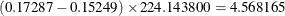The difference between the first and third R square times the total sum of squares is the model sum of squares for spline(x2):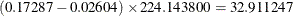Figure 97.71 displays the PROC REG results. The TEST statement in PROC REG tests the null hypothesis that the vector of parameters for x1_1 x1_2 x1_3 is zero. This is the same test as the spline(x1) test used by PROC TRANSREG. Similarly, the PROC REG test that the vector of parameters for x2_1 x2_2 x2_3 is zero is the same as the PROC TRANSREG SPLINE(x2) test. So for models with no monotonicity constraints and no dependent variable transformations, PROC TRANSREG provides little more than a different packaging of standard least squares methodology.

Figure 97.71: Two-Variable Polynomial Regression Output from PROC REG

 Two-Variable Polynomial Regression

The REG Procedure
Model: MODEL1
Dependent Variable: y

 Number of Observations Read 20 20

Analysis of Variance
Source DF Sum of
Squares
Mean
Square
F Value Pr > F
Model 6 38.74775 6.45796 0.45 0.8306
Error 13 185.39605 14.26123
Corrected Total 19 224.14380

 Root MSE R-Square 3.7764 0.1729 0.8549 -0.2089 441.734

Parameter Estimates
Variable Label DF Parameter
Estimate
Standard
Error
t Value Pr > |t|
Intercept Intercept 1 10.77824 7.55244 1.43 0.1771
x1_1 x1 1 1 0.40112 1.81024 0.22 0.8281
x1_2 x1 2 1 0.25652 1.66023 0.15 0.8796
x1_3 x1 3 1 -0.11639 0.36775 -0.32 0.7567
x2_1 x2 1 1 -14.07054 12.50521 -1.13 0.2809
x2_2 x2 2 1 5.95610 5.97952 1.00 0.3374
x2_3 x2 3 1 -0.80608 0.87291 -0.92 0.3726

 Two-Variable Polynomial Regression

The REG Procedure
Model: MODEL1

Test 1 Results for Dependent Variable y
Source DF Mean
Square
F Value Pr > F
Numerator 3 1.52272 0.11 0.9546
Denominator 13 14.26123

 Two-Variable Polynomial Regression

The REG Procedure
Model: MODEL1

Test 2 Results for Dependent Variable y
Source DF Mean
Square
F Value Pr > F
Numerator 3 10.97042 0.77 0.5315
Denominator 13 14.26123